# 处理缺失值的三个层级的方法总结

## 缺失值

• 初级:删除，均值/中值插补，使用领域知识进行估计
• 中级:回归插补, K-Nearest neighbors (KNN) 插补
• 高级:链式方程(MICE)的多元插补, MICEforest

## 检查缺失的值

`````` importpandasaspd

`` df.isnull().sum()``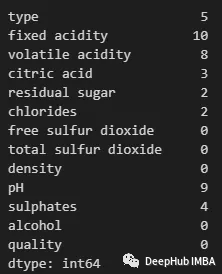`````` df_filtered=df[df.isnull().any(axis=1)]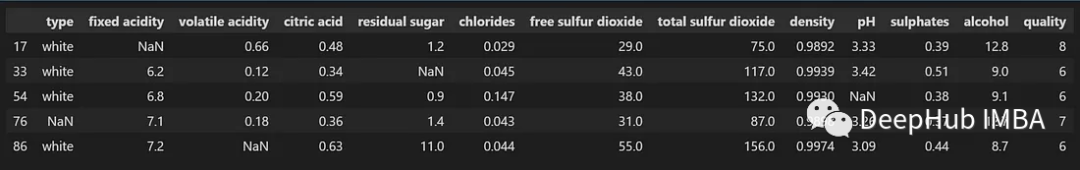## 初级方法

`````` df_droprows=df.dropna()
df_droprows.isnull().sum()``````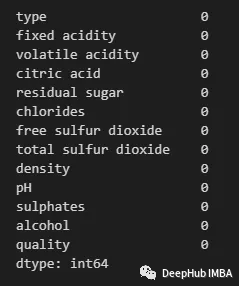`````` df_dropcols=df.drop(columns=['type', 'fixed acidity', 'citric acid', 'volatile acidity', 'residual sugar', 'chlorides', 'pH', 'sulphates'])
df_dropcols.isnull().sum()``````

`````` print("Shape when dropping rows: ", df_droprows.shape)
print("Shape when dropping features: ", df_dropcols.shape)``````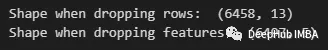`` df=df.fillna(df.mean())``

`` df[df.index==86]``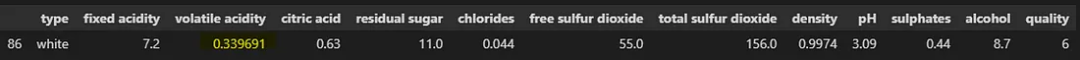`````` df = df.fillna(df.median())
df[df.index==86]``````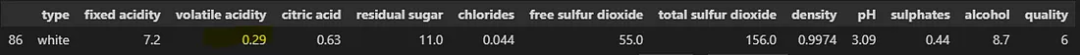`` df['type'].value_counts()``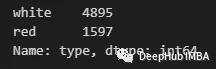`` df['type'] =df['type'].fillna(df['type'].mode())``

Scikit-Learn的SimpleImputer类

`````` df_numeric=df.drop(columns='type')
imputer_median=SimpleImputer(strategy='median')
imputer_median.fit(df_numeric)
df_imputed_median=pd.DataFrame(imputer_median.transform(df_numeric), columns=df_numeric.columns)

• 简单和快速实现
• 它保留了样本量，并降低了下游分析(如机器学习模型)的偏差风险。
• 与更复杂的方法相比，它的计算成本更低。

• 没有说明数据的可变性或分布，可能会导致估算值不能代表真实值。
• 可能会低估或高估缺失值，特别是在具有极端值或异常值的数据集中。
• 减少方差和人为夸大相关系数在估算数据集。
• 它假设缺失的值是完全随机缺失(MCAR)，这可能并不总是这样

## 中级方法

`````` importpandasaspd
fromsklearn.linear_modelimportLinearRegression

# Make sub dataframe with only numeric features
df=df.drop(columns='type')

# Separate the columns with missing values
missing_cols=df.columns[df.isna().any()].tolist()
non_missing_cols=list(set(df.columns) -set(missing_cols))

print(missing_cols)

# loop over each column with missing values
forcolinmissing_cols:
# Create a copy of the dataframe without missing values in the current column
df_temp=df.dropna(subset=[col] +non_missing_cols)

# Split the dataframe into features (X) and target variable (y)
X=df_temp[non_missing_cols]
y=df_temp[col]

# Create and fit a linear regression model
lr=LinearRegression()
lr.fit(X, y)

# Impute missing values in the current column using the fitted model
df.loc[df[col].isna(), col] =lr.predict(df.loc[df[col].isna(), non_missing_cols])``````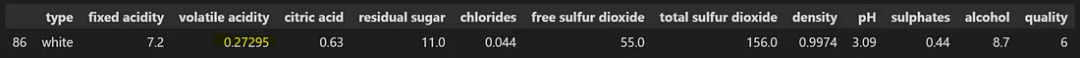• 可以处理大量缺失值。
• 可以保留数据集的统计属性，例如均值、方差和相关系数。
• 可以通过减少偏差和增加样本量来提高下游分析（例如机器学习模型）的准确性。

• 它假设缺失变量和观察到的变量之间存在线性关系。
• 如果缺失值不是随机缺失 (MAR) 或完全随机缺失 (MCAR)，则可能会引入偏差。
• 可能不适用于分类或有序变量。
• 在计算上昂贵且耗时，尤其是对于大型数据集。

(KNN) 插补

`````` importpandasaspd
fromsklearn.imputeimportKNNImputer

# Make sub dataframe with only numeric features
df=df.drop(columns='type')

# create a KNN imputer object
imputer=KNNImputer(n_neighbors=5)

# impute missing values using KNN
df=pd.DataFrame(imputer.fit_transform(df), columns=df.columns)``````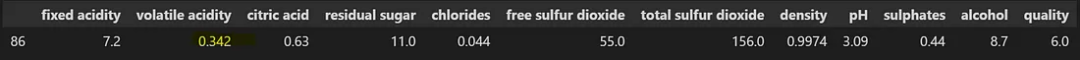`` pip install fancyimpute``

`````` # Import the necessary libraries
importnumpyasnp
importpandasaspd
fromfancyimputeimportKNN

# Drop non-numeric features
df=df.drop(columns='type')

# Get list of columns with missing values
missing_cols=df.columns[df.isna().any()].tolist()

# Create an instance of the KNN imputer
imputer=KNN()

# Fit and transform the imputer to the dataset
imputed_array=imputer.fit_transform(df[missing_cols])

# Replace the missing values in the original dataset
df[missing_cols] =imputed_array

# View the imputed dataset
df``````

KNN 插补的优点：

• 可以捕获变量之间复杂的非线性关系。
• 不对数据的分布或变量之间的相关性做出假设。
• 比简单的插补方法（例如均值或中值插补）更准确，尤其是对于中小型数据集。

• 计算上可能很昂贵，尤其是对于大型数据集或高维数据。
• 可能对距离度量的选择和选择的最近邻居的数量敏感，这会影响准确性。
• 对于高度倾斜或稀疏的数据表现不佳。

## 高级方法

MICE 是一种常用的估算缺失数据的方法。它的工作原理是将每个缺失值替换为一组基于模型的合理值，该模型考虑了数据集中变量之间的关系。

fancyimpute包就包含了这个方法的实现，我们可以直接拿来使用

`````` importnumpyasnp
importpandasaspd
fromfancyimputeimportIterativeImputer

# Convert type column to category (so that miceforest can handle as a categorical attribute rather than string)
df=df.drop(columns='type')

# Get list of columns with missing values
missing_cols=df.columns[df.isna().any()].tolist()

# Create an instance of the MICE algorithm
imputer=IterativeImputer()

# Fit the imputer to the dataset
imputed_array=imputer.fit_transform(df[missing_cols])

# Replace the missing values in the original dataset
df[missing_cols] =imputed_array

# View the imputed dataset
df``````

MICEforest

MICEforest 是 MICE的变体，它使用 lightGBM 算法来插补数据集中的缺失值，这是一个很奇特的想法，对吧。

`````` pipinstallmiceforest
#或
condainstall-cconda-forgemiceforest``````

`````` importpandasaspd
importmiceforestasmf

# Convert type column to category (so that miceforest can handle as a categorical attribute rather than string)
df['type'] =df['type'].astype('category')

# Create an instance of the MICE algorithm
imputer=mf.ImputationKernel(data=df,
save_all_iterations=True,
random_state=42)

# Fit the imputer to the dataset. Set number of iterations to 3
imputer.mice(3, verbose=True)

# Generate the imputed dataset
imputed_df=imputer.complete_data()

# View the imputed dataset
imputed_df``````

## 总结

https://avoid.overfit.cn/post/803b18d975bc4cdd82aa3026cd405ce8

1 阅读 122
##### 推荐阅读3959

460
SegmentFault 思否旗下人工智能领域产业媒体，专注技术与产业，一起探索人工智能。